Study Materials

# NCERT Solutions for Class 11th Mathematics

Page 1 of 5

## Exercise 9.1

Write the first five terms of each of the sequences in Exercises 1 to 6 whose nth terms are: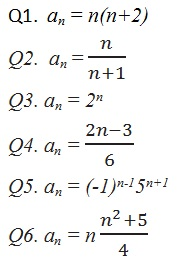Q1. Write the first five terms of the sequences whose nth term is an = n(n+2).

Solution: Q1.

is an = n(n+2)

Putting n = 1, 2, 3, 4 and 5 we obtain

a1 = 1(1 + 2) = 1× 3 = 3

a2 = 2(2 + 2) = 2× 4 = 8

a3 = 3(3 + 2) = 3× 5 = 15

a4 = 4(4 + 2) = 4× 6 = 24

a5 = 5(5 + 2) = 5× 7 = 35

Therefore, the five terms are 3, 8, 15, 24 and 35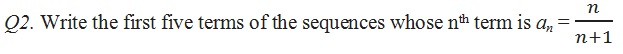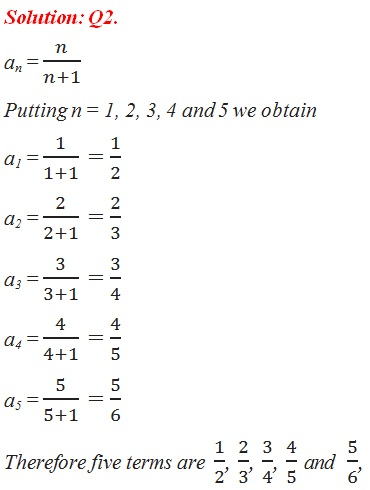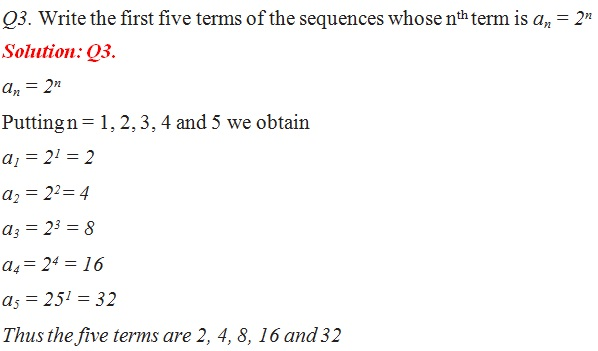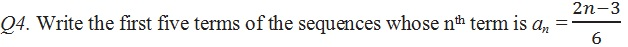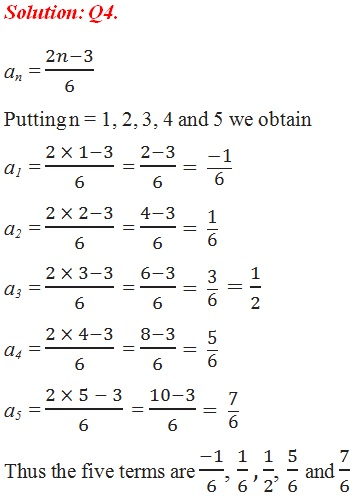Q5. Write the first five terms of the sequences whose nth term is an = (-1)n-15n+1

Solution: Q5.

an = (-1)n-15n+1

Putting n = 1, 2, 3, 4 and 5 we obtain

a1 = (-1)1-151+1 = (1)(5)2 = 25

a2 = (-1)2-152+1 = (-1) (5)3 = - 125

a3 = (-1)3-153+1 = (1) (5)4 = 625

a4 = (-1)4-154+1 = (-1) (5)5 = - 3125

a5 = (-1)5-155+1 = (1) (5)6 =156 25

Thus the five terms are 25, -125, 625, - 3125 and 15625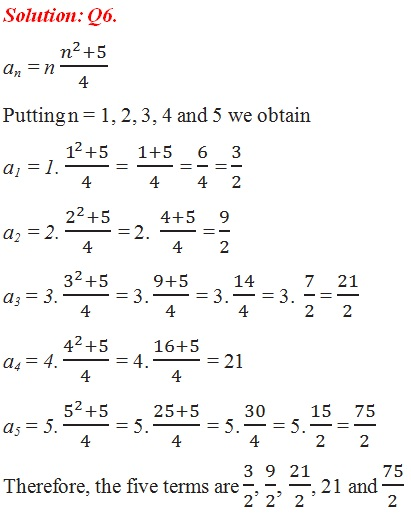Page 1 of 5

Chapter Contents: